Next: Ideal Gas Equation of Up: Classical Thermodynamics Previous: Classical Thermodynamics

# Introduction

We have already learned that macroscopic quantities, such as energy, temperature, and pressure, are, in fact, statistical in nature. In other words, in an equilibrium state, they exhibit random fluctuations about some mean value. If we were to plot the probability distribution for the energy (say) of a system in thermal equilibrium with its surroundings then we would obtain a Gaussian distribution with a very small fractional width. In fact, we expect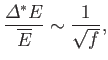(6.1)

where the number of degrees of freedom,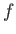, is about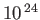for laboratory-scale systems. This implies that the statistical fluctuations of macroscopic quantities about their mean values are typically only about 1 part in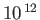.

Because the statistical fluctuations of equilibrium quantities are so small, it is an excellent approximation to neglect them altogether, and, thereby, to replace macroscopic quantities, such as energy, temperature, and pressure, by their mean values. In other words,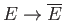,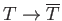,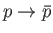, et cetera. In the following discussion, we shall drop the overbars, so that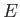should be understood to represent the mean energy,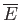, et cetera. This prescription, which is the essence of classical thermodynamics, is equivalent to replacing all statistically-varying quantities by their most probable values.

Although, formally, there are four laws of thermodynamics (i.e., the zeroth to the third), the zeroth law is really a consequence of the second law, and the third law is only important at temperatures close to absolute zero. So, for most practical purposes, the two laws that actually matter are the first law and the second law.

For an infinitesimal process, the first law of thermodynamics is written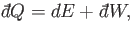(6.2)

where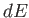is the change in internal energy of the system,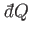the heat absorbed by the system from its surroundings, and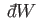the work done by the system on its surroundings. Note that this particular formulation of the first law is merely a convention. We could equally well write the first law in terms of the heat emitted by the system, or the work done on the system. It does not really matter, as long as we are consistent in our definitions. The second law of thermodynamics implies that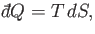(6.3)

for an infinitesimal quasi-static process, whereis the thermodynamic temperature, and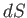the change in entropy of the system. Furthermore, for systems in which the only external parameter is the volume (e.g., gases), the work done on the environment is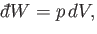(6.4)

whereis the pressure, and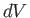the change in volume. Thus, the first and second laws of thermodynamics can be combined to give the fundamental thermodynamic relation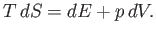(6.5)Next: Ideal Gas Equation of Up: Classical Thermodynamics Previous: Classical Thermodynamics
Richard Fitzpatrick 2016-01-25